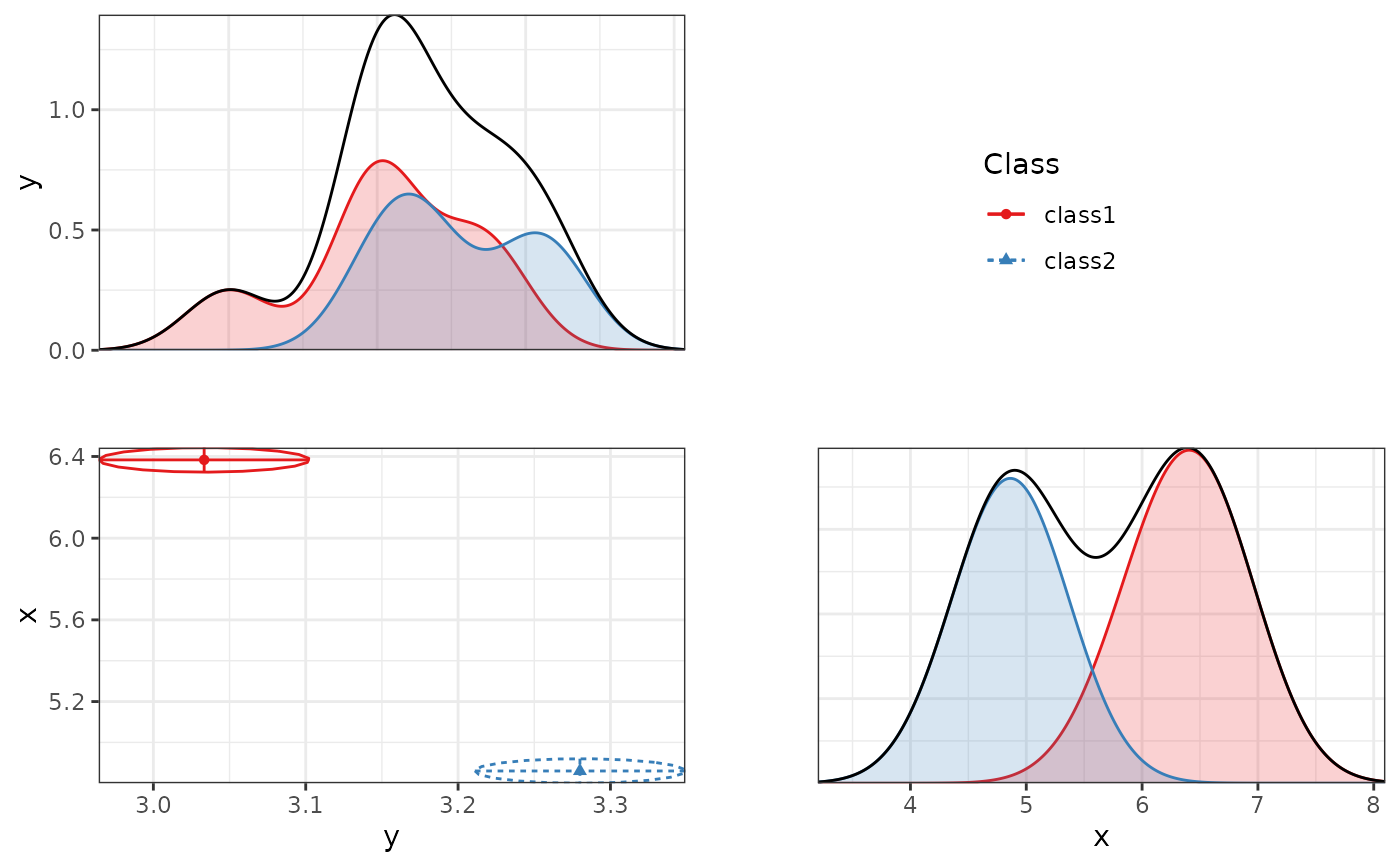Creates a faceted plot of two-dimensional correlation plots and unidimensional density plots for a single mixture model.

plot_bivariate(
x,
variables = NULL,
sd = TRUE,
cors = TRUE,
rawdata = TRUE,
bw = FALSE,
alpha_range = c(0, 0.1),
return_list = FALSE
)

## Arguments

x

An object for which a method exists.

variables

Which variables to plot. If NULL, plots all variables that are present in the model.

sd

Logical. Whether to show the estimated standard deviations as lines emanating from the cluster centroid.

cors

Logical. Whether to show the estimated correlation (standardized covariance) as ellipses surrounding the cluster centroid.

rawdata

Logical. Whether to plot raw data, weighted by posterior class probability.

bw

Logical. Whether to make a black and white plot (for print) or a color plot. Defaults to FALSE, because these density plots are hard to read in black and white.

alpha_range

Numeric vector (0-1). Sets the transparency of geom_density and geom_point.

return_list

Logical. Whether to return a list of ggplot objects, or just the final plot. Defaults to FALSE.

## Value

An object of class 'ggplot'.

## Author

Caspar J. van Lissa

## Examples

iris_sample <- iris[c(1:5, 145:150), c("Sepal.Length", "Sepal.Width")]
names(iris_sample) <- c("x", "y")
res <- mx_profiles(iris_sample, classes = 2)
#> Running mix2 with 7 parameters
#> Running mix2 with 7 parameters
plot_bivariate(res, rawdata = FALSE)